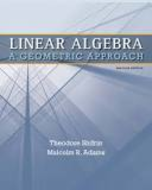Linear Algebra: A Geometric Approach - 2 Edition - Chapter 1.4 - Problem 16
Register Now

Join StudySoup

Get Full Access to Linear Algebra: A Geometric Approach - 2 Edition - Chapter 1.4 - Problem 169781429215213

# Prove that the reduced echelon form of a matrix is unique, as follows. Suppose B and C

Linear Algebra: A Geometric Approach | 2nd Edition

Problem 16

Prove that the reduced echelon form of a matrix is unique, as follows. Suppose B and C are reduced echelon forms of a given nonzero $$m \times n$$ matrix A.

a. Deduce from the proof of Proposition 4.2 that B andC have the same pivot variables.

b. Explain why the pivots of B and C are in the identical positions. (This is true even without the assumption that the matrices are in reduced echelon form.)

c. By considering the solutions in standard form of Bx = 0 and Cx = 0, deduce that B = C.

Accepted Solution
Step-by-Step Solution:

Step 1 of 4

It is given that,andare the reduced echelon forms of a given nonzeromatrix.

It is known that every leading entry isfor the reduced echelon form.

Also, all the entries of the column above each leading entry are.

To prove that the reduced echelon form of a matrix is unique.

###### Chapter 1.4, Problem 16 is Solved

Step 2 of 4

Step 3 of 4

Unlock Textbook Solution